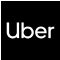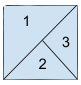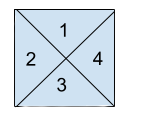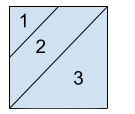New update is available. Click here to update.
Topics

# Regions Cut By Slashes

Moderate0/80
Average time to solve is 30m
0 upvote+1 more companies

## Problem statement

This time our favorite Ninja wants to buy a house. But they are unable to count the number of rooms in the house. So they need your help to count the number of rooms.

Note:
``````A house is represented by a matrix where “\” and “/” represent a wall and blank space is empty space. We will consider two rooms different if we will be unable to reach another room.
``````

Your task is to return the number of rooms. For a better explanation, see the example.

Example:
``````Input:
[
“ /”
“/\”
]

Output: 3

Explanation: 2 X 2 house is shown below:
``````Detailed explanation ( Input/output format, Notes, Images )
Constraints:
``````1 <= T <= 5
2 <= N <= 30

Time limit: 1 sec
``````
Sample Input 1:
``````2
2
\/
/\
3
/ /
/
/
``````
Sample Output 1:
``````4
3
``````
Explanation of Sample Output 1:
``````In test case 1, We can see there are 4 separate rooms.
````````````In test case 2, we can see there are 3 separate rooms.
``````Sample Input 2:
``````1
2
/
/\
``````

#### Sample Output 2:

``````3
``````
Explanation of Sample Output 2:
``````In test case 1, We can see there are 4 separate rooms.
``````Console